# chapt20_lecture.ppt

Mar. 22, 2023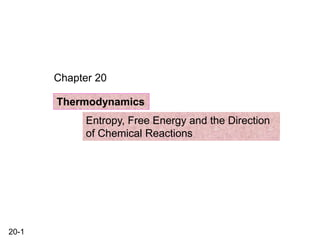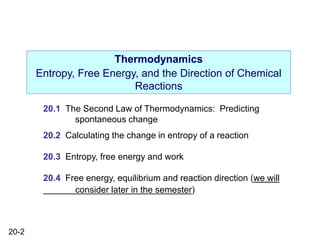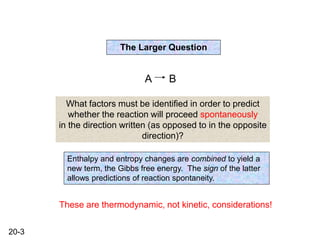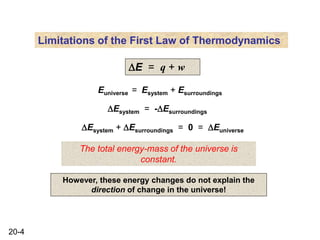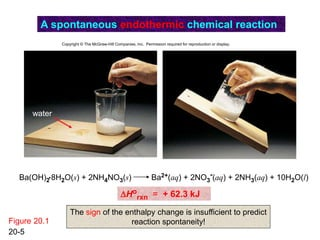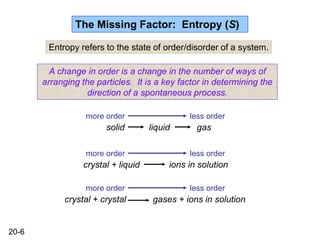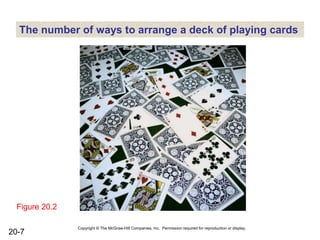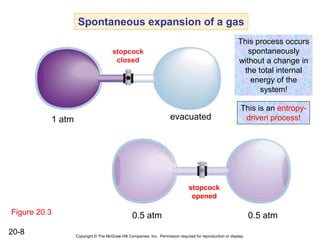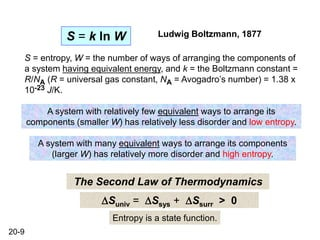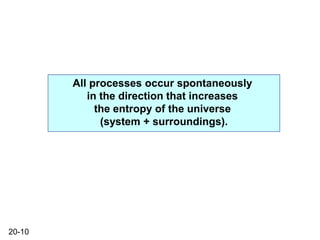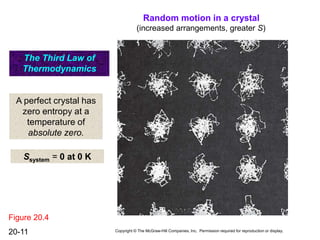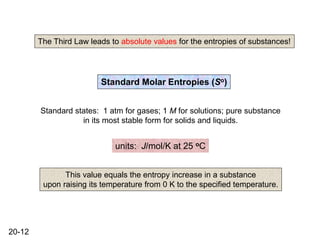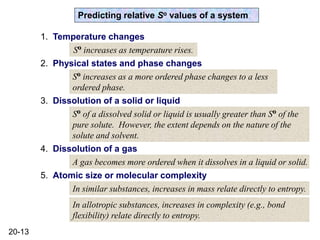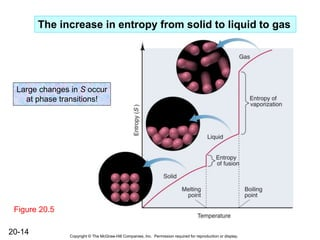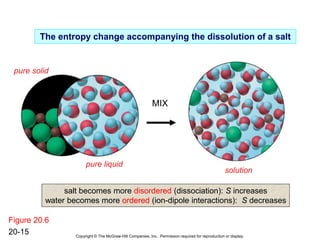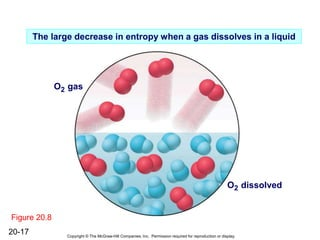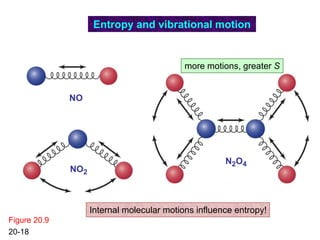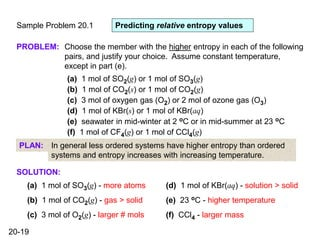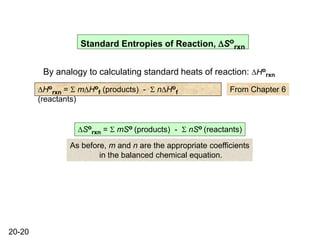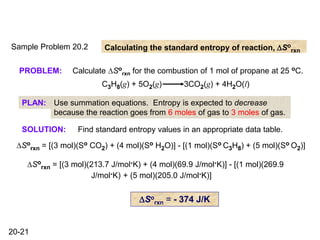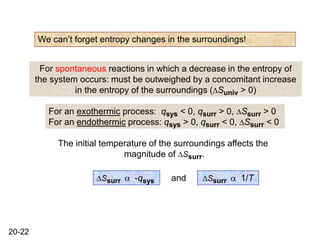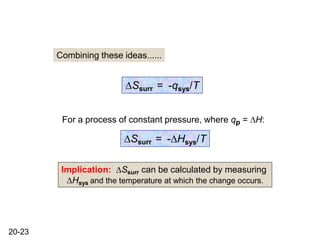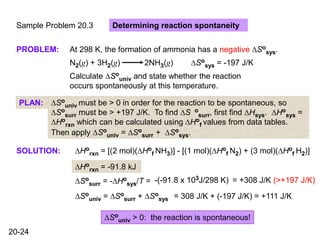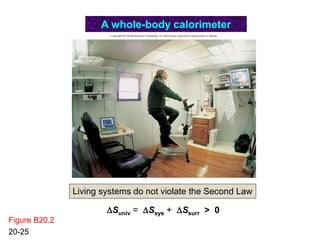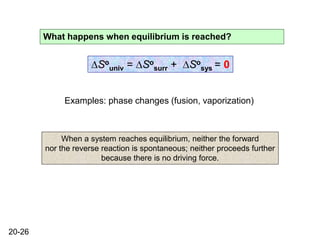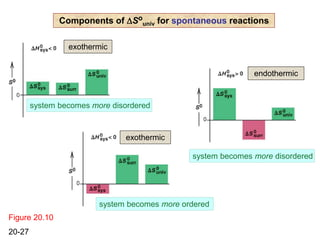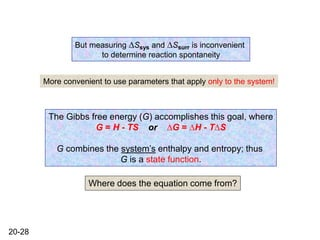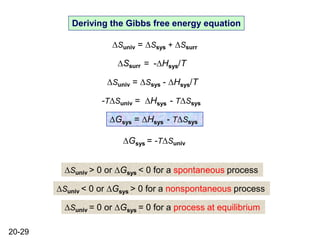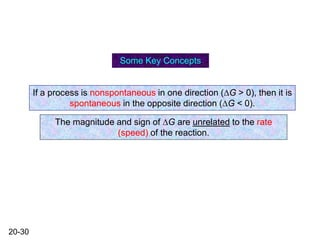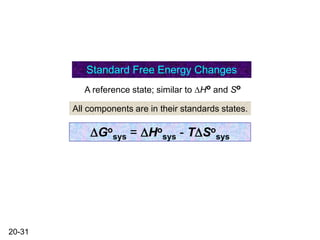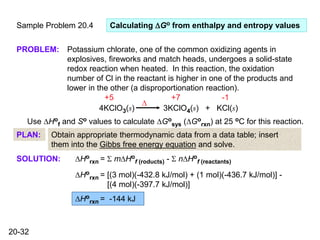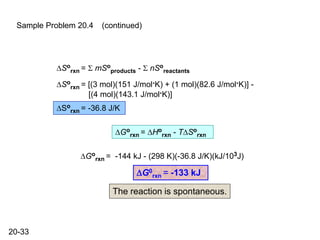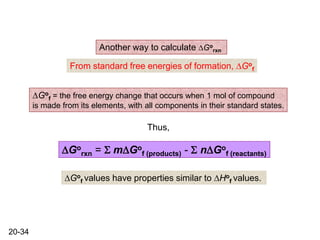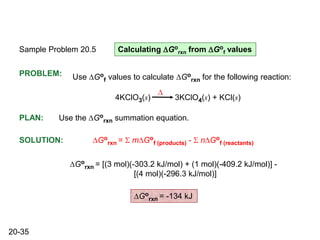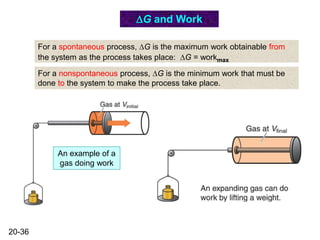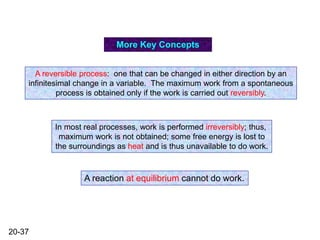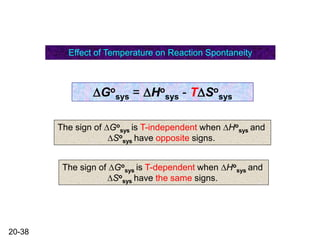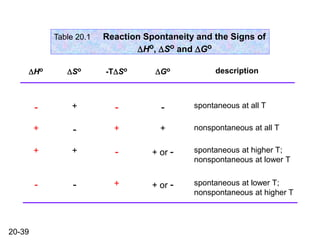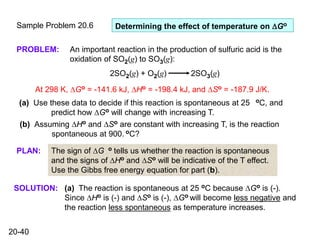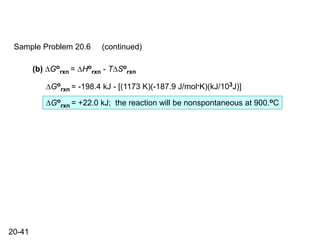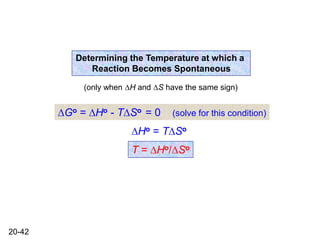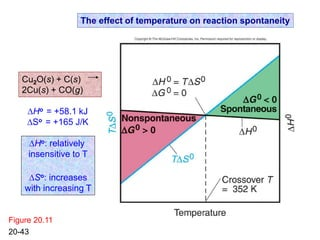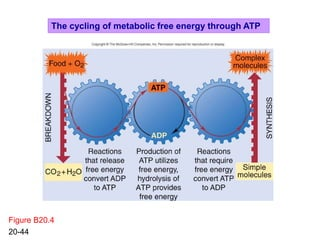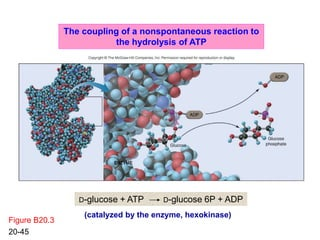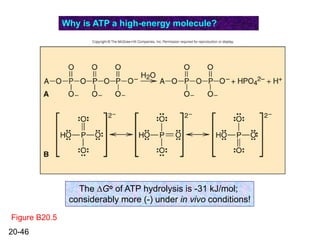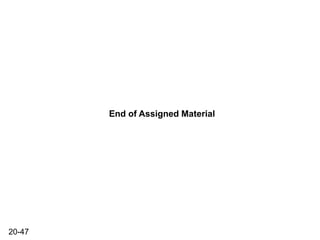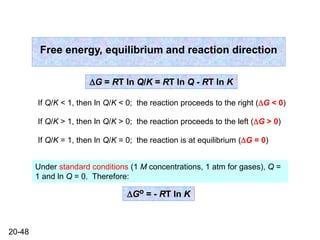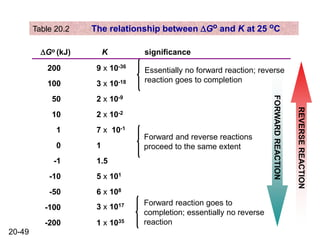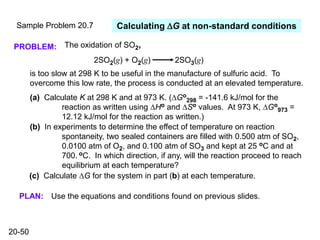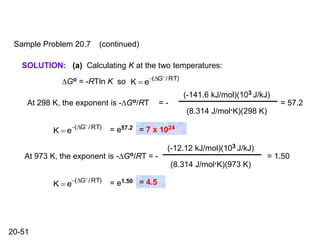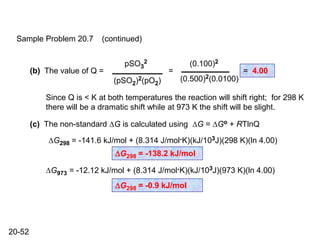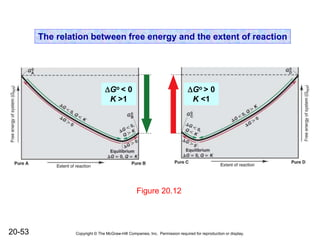1 of 53

### chapt20_lecture.ppt

1. 20-1 Chapter 20 Thermodynamics Entropy, Free Energy and the Direction of Chemical Reactions
2. 20-2 Thermodynamics Entropy, Free Energy, and the Direction of Chemical Reactions 20.1 The Second Law of Thermodynamics: Predicting spontaneous change 20.2 Calculating the change in entropy of a reaction 20.3 Entropy, free energy and work 20.4 Free energy, equilibrium and reaction direction (we will consider later in the semester)
3. 20-3 The Larger Question A B What factors must be identified in order to predict whether the reaction will proceed spontaneously in the direction written (as opposed to in the opposite direction)? Enthalpy and entropy changes are combined to yield a new term, the Gibbs free energy. The sign of the latter allows predictions of reaction spontaneity. These are thermodynamic, not kinetic, considerations!
4. 20-4 Limitations of the First Law of Thermodynamics DE = q + w Euniverse = Esystem + Esurroundings DEsystem = -DEsurroundings The total energy-mass of the universe is constant. However, these energy changes do not explain the direction of change in the universe! DEsystem + DEsurroundings = 0 = DEuniverse
5. 20-5 Figure 20.1 A spontaneous endothermic chemical reaction water Ba(OH)2 8H2O(s) + 2NH4NO3(s) Ba2+(aq) + 2NO3 -(aq) + 2NH3(aq) + 10H2O(l) . DHo rxn = + 62.3 kJ Copyright © The McGraw-Hill Companies, Inc. Permission required for reproduction or display. The sign of the enthalpy change is insufficient to predict reaction spontaneity!
6. 20-6 The Missing Factor: Entropy (S) Entropy refers to the state of order/disorder of a system. A change in order is a change in the number of ways of arranging the particles. It is a key factor in determining the direction of a spontaneous process. solid liquid gas more order less order crystal + liquid ions in solution more order less order more order less order crystal + crystal gases + ions in solution
7. 20-7 Figure 20.2 The number of ways to arrange a deck of playing cards Copyright © The McGraw-Hill Companies, Inc. Permission required for reproduction or display.
8. 20-8 Figure 20.3 1 atm evacuated Spontaneous expansion of a gas stopcock closed stopcock opened 0.5 atm 0.5 atm Copyright © The McGraw-Hill Companies, Inc. Permission required for reproduction or display. This process occurs spontaneously without a change in the total internal energy of the system! This is an entropy- driven process!
9. 20-9 Ludwig Boltzmann, 1877 S = k ln W S = entropy, W = the number of ways of arranging the components of a system having equivalent energy, and k = the Boltzmann constant = R/NA (R = universal gas constant, NA = Avogadro’s number) = 1.38 x 10-23 J/K. A system with relatively few equivalent ways to arrange its components (smaller W) has relatively less disorder and low entropy. A system with many equivalent ways to arrange its components (larger W) has relatively more disorder and high entropy. DSuniv = DSsys + DSsurr > 0 The Second Law of Thermodynamics Entropy is a state function.
10. 20-10 All processes occur spontaneously in the direction that increases the entropy of the universe (system + surroundings).
11. 20-11 Random motion in a crystal The Third Law of Thermodynamics A perfect crystal has zero entropy at a temperature of absolute zero. Ssystem = 0 at 0 K Copyright © The McGraw-Hill Companies, Inc. Permission required for reproduction or display. Figure 20.4 (increased arrangements, greater S)
12. 20-12 Standard Molar Entropies (So) Standard states: 1 atm for gases; 1 M for solutions; pure substance in its most stable form for solids and liquids. units: J/mol/K at 25 oC The Third Law leads to absolute values for the entropies of substances! This value equals the entropy increase in a substance upon raising its temperature from 0 K to the specified temperature.
13. 20-13 Predicting relative So values of a system 1. Temperature changes 2. Physical states and phase changes 3. Dissolution of a solid or liquid 5. Atomic size or molecular complexity 4. Dissolution of a gas So increases as temperature rises. So increases as a more ordered phase changes to a less ordered phase. So of a dissolved solid or liquid is usually greater than So of the pure solute. However, the extent depends on the nature of the solute and solvent. A gas becomes more ordered when it dissolves in a liquid or solid. In similar substances, increases in mass relate directly to entropy. In allotropic substances, increases in complexity (e.g., bond flexibility) relate directly to entropy.
14. 20-14 Figure 20.5 The increase in entropy from solid to liquid to gas Copyright © The McGraw-Hill Companies, Inc. Permission required for reproduction or display. Large changes in S occur at phase transitions!
15. 20-15 Figure 20.6 The entropy change accompanying the dissolution of a salt pure solid pure liquid solution MIX Copyright © The McGraw-Hill Companies, Inc. Permission required for reproduction or display. salt becomes more disordered (dissociation): S increases water becomes more ordered (ion-dipole interactions): S decreases
16. 20-16 Figure 20.7 ethanol water solution of ethanol and water The small increase in entropy when ethanol dissolves in water Copyright © The McGraw-Hill Companies, Inc. Permission required for reproduction or display. Freedom of movement remains essentially unchanged; S increase is due solely to random mixing effects.
17. 20-17 Figure 20.8 The large decrease in entropy when a gas dissolves in a liquid O2 gas Copyright © The McGraw-Hill Companies, Inc. Permission required for reproduction or display. O2 dissolved
18. 20-18 Figure 20.9 NO NO2 N2O4 Entropy and vibrational motion Internal molecular motions influence entropy! more motions, greater S
19. 20-19 Sample Problem 20.1 SOLUTION: Predicting relative entropy values PROBLEM: Choose the member with the higher entropy in each of the following pairs, and justify your choice. Assume constant temperature, except in part (e). (a) 1 mol of SO2(g) or 1 mol of SO3(g) (b) 1 mol of CO2(s) or 1 mol of CO2(g) (c) 3 mol of oxygen gas (O2) or 2 mol of ozone gas (O3) (d) 1 mol of KBr(s) or 1 mol of KBr(aq) (e) seawater in mid-winter at 2 oC or in mid-summer at 23 oC (f) 1 mol of CF4(g) or 1 mol of CCl4(g) PLAN: In general less ordered systems have higher entropy than ordered systems and entropy increases with increasing temperature. (a) 1 mol of SO3(g) - more atoms (b) 1 mol of CO2(g) - gas > solid (c) 3 mol of O2(g) - larger # mols (d) 1 mol of KBr(aq) - solution > solid (e) 23 oC - higher temperature (f) CCl4 - larger mass
20. 20-20 Standard Entropies of Reaction, DSo rxn By analogy to calculating standard heats of reaction: DHo rxn DHo rxn = S mDHo f (products) - S nDHo f (reactants) DSo rxn = S mSo (products) - S nSo (reactants) From Chapter 6 As before, m and n are the appropriate coefficients in the balanced chemical equation.
21. 20-21 Sample Problem 20.2 Calculating the standard entropy of reaction, DSo rxn PROBLEM: Calculate DSo rxn for the combustion of 1 mol of propane at 25 oC. C3H8(g) + 5O2(g) 3CO2(g) + 4H2O(l) PLAN: Use summation equations. Entropy is expected to decrease because the reaction goes from 6 moles of gas to 3 moles of gas. SOLUTION: Find standard entropy values in an appropriate data table. DSo rxn = [(3 mol)(So CO2) + (4 mol)(So H2O)] - [(1 mol)(So C3H8) + (5 mol)(So O2)] DSo rxn = [(3 mol)(213.7 J/mol.K) + (4 mol)(69.9 J/mol.K)] - [(1 mol)(269.9 J/mol.K) + (5 mol)(205.0 J/mol.K)] DSo rxn = - 374 J/K
22. 20-22 We can’t forget entropy changes in the surroundings! For spontaneous reactions in which a decrease in the entropy of the system occurs: must be outweighed by a concomitant increase in the entropy of the surroundings (DSuniv > 0) For an exothermic process: qsys < 0, qsurr > 0, DSsurr > 0 For an endothermic process: qsys > 0, qsurr < 0, DSsurr < 0 The initial temperature of the surroundings affects the magnitude of DSsurr. DSsurr a -qsys DSsurr a 1/T and
23. 20-23 DSsurr = -qsys/T For a process of constant pressure, where qp = DH: DSsurr = -DHsys/T Implication: DSsurr can be calculated by measuring DHsys and the temperature at which the change occurs. Combining these ideas......
24. 20-24 Sample Problem 20.3 SOLUTION: Determining reaction spontaneity PROBLEM: At 298 K, the formation of ammonia has a negative DSo sys. Calculate DSo univ and state whether the reaction occurs spontaneously at this temperature. N2(g) + 3H2(g) 2NH3(g) DSo sys = -197 J/K PLAN: DSo univ must be > 0 in order for the reaction to be spontaneous, so DSo surr must be > +197 J/K. To find DS o surr, first find DHsys. DHo sys = DHo rxn which can be calculated using DHo f values from data tables. Then apply DSo univ = DSo surr + DSo sys. DHo rxn = [(2 mol)(DHo f NH3)] - [(1 mol)(DHo f N2) + (3 mol)(DHo f H2)] DHo rxn = -91.8 kJ DSo surr = -DHo sys/T = -(-91.8 x 103J/298 K) = +308 J/K (>+197 J/K) DSo univ = DSo surr + DSo sys = 308 J/K + (-197 J/K) = +111 J/K DSo univ > 0: the reaction is spontaneous!
25. 20-25 Figure B20.2 A whole-body calorimeter Living systems do not violate the Second Law DSuniv = DSsys + DSsurr > 0
26. 20-26 What happens when equilibrium is reached? DSo univ = DSo surr + DSo sys = 0 Examples: phase changes (fusion, vaporization) When a system reaches equilibrium, neither the forward nor the reverse reaction is spontaneous; neither proceeds further because there is no driving force.
27. 20-27 Figure 20.10 Components of DSo univ for spontaneous reactions exothermic system becomes more disordered exothermic system becomes more ordered endothermic system becomes more disordered
28. 20-28 But measuring DSsys and DSsurr is inconvenient to determine reaction spontaneity More convenient to use parameters that apply only to the system! The Gibbs free energy (G) accomplishes this goal, where G = H - TS or DG = DH - TDS G combines the system’s enthalpy and entropy; thus G is a state function. Where does the equation come from?
29. 20-29 Deriving the Gibbs free energy equation DSuniv = DSsys + DSsurr DSsurr = -DHsys/T DSuniv = DSsys - DHsys/T -TDSuniv = DHsys - TDSsys DGsys = DHsys - TDSsys DGsys = -TDSuniv DSuniv > 0 or DGsys < 0 for a spontaneous process DSuniv < 0 or DGsys > 0 for a nonspontaneous process DSuniv = 0 or DGsys = 0 for a process at equilibrium
30. 20-30 If a process is nonspontaneous in one direction (DG > 0), then it is spontaneous in the opposite direction (DG < 0). The magnitude and sign of DG are unrelated to the rate (speed) of the reaction. Some Key Concepts
31. 20-31 DGo sys = DHo sys - TDSo sys Standard Free Energy Changes All components are in their standards states. A reference state; similar to DHo and So
32. 20-32 Sample Problem 20.4 SOLUTION: Calculating DGo from enthalpy and entropy values PROBLEM: Potassium chlorate, one of the common oxidizing agents in explosives, fireworks and match heads, undergoes a solid-state redox reaction when heated. In this reaction, the oxidation number of Cl in the reactant is higher in one of the products and lower in the other (a disproportionation reaction). 4KClO3(s) 3KClO4(s) + KCl(s) D +7 -1 +5 Use DHo f and So values to calculate DGo sys (DGo rxn) at 25 oC for this reaction. PLAN: Obtain appropriate thermodynamic data from a data table; insert them into the Gibbs free energy equation and solve. DHo rxn = S mDHo f (roducts) - S nDHo f (reactants) DHo rxn = [(3 mol)(-432.8 kJ/mol) + (1 mol)(-436.7 kJ/mol)] - [(4 mol)(-397.7 kJ/mol)] DHo rxn = -144 kJ
33. 20-33 Sample Problem 20.4 (continued) DSo rxn = S mSo products - S nSo reactants DSo rxn = [(3 mol)(151 J/mol.K) + (1 mol)(82.6 J/mol.K)] - [(4 mol)(143.1 J/mol.K)] DSo rxn = -36.8 J/K DGo rxn = DHo rxn - TDSo rxn DGo rxn = -144 kJ - (298 K)(-36.8 J/K)(kJ/103J) DG0 rxn = -133 kJ The reaction is spontaneous.
34. 20-34 DGo rxn = S mDGo f (products) - S nDGo f (reactants) Another way to calculate DGo rxn From standard free energies of formation, DGo f DGo f = the free energy change that occurs when 1 mol of compound is made from its elements, with all components in their standard states. Thus, DGo f values have properties similar to DHo f values.
35. 20-35 Sample Problem 20.5 PROBLEM: Calculating DGo rxn from DGo f values Use DGo f values to calculate DGo rxn for the following reaction: 4KClO3(s) 3KClO4(s) + KCl(s) D PLAN: Use the DGo rxn summation equation. SOLUTION: DGo rxn = S mDGo f (products) - S nDGo f (reactants) DGo rxn = [(3 mol)(-303.2 kJ/mol) + (1 mol)(-409.2 kJ/mol)] - [(4 mol)(-296.3 kJ/mol)] DGo rxn = -134 kJ
36. 20-36 DG and Work For a spontaneous process, DG is the maximum work obtainable from the system as the process takes place: DG = workmax For a nonspontaneous process, DG is the minimum work that must be done to the system to make the process take place. An example of a gas doing work
37. 20-37 A reversible process: one that can be changed in either direction by an infinitesimal change in a variable. The maximum work from a spontaneous process is obtained only if the work is carried out reversibly. In most real processes, work is performed irreversibly; thus, maximum work is not obtained; some free energy is lost to the surroundings as heat and is thus unavailable to do work. A reaction at equilibrium cannot do work. More Key Concepts
38. 20-38 Effect of Temperature on Reaction Spontaneity DGo sys = DHo sys - TDSo sys The sign of DGo sys is T-independent when DHo sys and DSo sys have opposite signs. The sign of DGo sys is T-dependent when DHo sys and DSo sys have the same signs.
39. 20-39 Table 20.1 Reaction Spontaneity and the Signs of DHo, DSo and DGo DHo DSo -TDSo DGo description - + - - + - + + + + - + or - - - + + or - spontaneous at all T nonspontaneous at all T spontaneous at higher T; nonspontaneous at lower T spontaneous at lower T; nonspontaneous at higher T
40. 20-40 Sample Problem 20.6 PROBLEM: PLAN: SOLUTION: Determining the effect of temperature on DGo An important reaction in the production of sulfuric acid is the oxidation of SO2(g) to SO3(g): 2SO2(g) + O2(g) 2SO3(g) At 298 K, DGo = -141.6 kJ, DHo = -198.4 kJ, and DSo = -187.9 J/K. (a) Use these data to decide if this reaction is spontaneous at 25 oC, and predict how DGo will change with increasing T. (b) Assuming DHo and DSo are constant with increasing T, is the reaction spontaneous at 900. oC? The sign of DG o tells us whether the reaction is spontaneous and the signs of DHo and DSo will be indicative of the T effect. Use the Gibbs free energy equation for part (b). (a) The reaction is spontaneous at 25 oC because DGo is (-). Since DHo is (-) and DSo is (-), DGo will become less negative and the reaction less spontaneous as temperature increases.
41. 20-41 Sample Problem 20.6 (continued) (b) DGo rxn = DHo rxn - TDSo rxn DGo rxn = -198.4 kJ - [(1173 K)(-187.9 J/mol.K)(kJ/103J)] DGo rxn = +22.0 kJ; the reaction will be nonspontaneous at 900.oC
42. 20-42 Determining the Temperature at which a Reaction Becomes Spontaneous (only when DH and DS have the same sign) DGo = DHo - TDSo = 0 (solve for this condition) DHo = TDSo T = DHo/DSo
43. 20-43 Figure 20.11 The effect of temperature on reaction spontaneity DHo: relatively insensitive to T DSo: increases with increasing T Cu2O(s) + C(s) 2Cu(s) + CO(g) DHo = +58.1 kJ DSo = +165 J/K
44. 20-44 Figure B20.4 The cycling of metabolic free energy through ATP
45. 20-45 Figure B20.3 The coupling of a nonspontaneous reaction to the hydrolysis of ATP D-glucose + ATP D-glucose 6P + ADP (catalyzed by the enzyme, hexokinase)
46. 20-46 Figure B20.5 Why is ATP a high-energy molecule? The DGo of ATP hydrolysis is -31 kJ/mol; considerably more (-) under in vivo conditions!
47. 20-47 End of Assigned Material
48. 20-48 Free energy, equilibrium and reaction direction If Q/K < 1, then ln Q/K < 0; the reaction proceeds to the right (DG < 0) If Q/K > 1, then ln Q/K > 0; the reaction proceeds to the left (DG > 0) If Q/K = 1, then ln Q/K = 0; the reaction is at equilibrium (DG = 0) DG = RT ln Q/K = RT ln Q - RT ln K Under standard conditions (1 M concentrations, 1 atm for gases), Q = 1 and ln Q = 0. Therefore: DGo = - RT ln K
49. 20-49 FORWARD REACTION REVERSE REACTION Table 20.2 The relationship between DGo and K at 25 oC DGo (kJ) K significance 200 100 50 10 1 0 -1 -10 -50 -100 -200 9 x 10-36 3 x 10-18 2 x 10-9 2 x 10-2 7 x 10-1 1 1.5 5 x 101 6 x 108 3 x 1017 1 x 1035 Essentially no forward reaction; reverse reaction goes to completion Forward and reverse reactions proceed to the same extent Forward reaction goes to completion; essentially no reverse reaction
50. 20-50 Sample Problem 20.7 PROBLEM: Calculating DG at non-standard conditions The oxidation of SO2, 2SO2(g) + O2(g) 2SO3(g) is too slow at 298 K to be useful in the manufacture of sulfuric acid. To overcome this low rate, the process is conducted at an elevated temperature. (a) Calculate K at 298 K and at 973 K. (DGo 298 = -141.6 kJ/mol for the reaction as written using DHo and DSo values. At 973 K, DGo 973 = 12.12 kJ/mol for the reaction as written.) (b) In experiments to determine the effect of temperature on reaction spontaneity, two sealed containers are filled with 0.500 atm of SO2, 0.0100 atm of O2, and 0.100 atm of SO3 and kept at 25 oC and at 700. oC. In which direction, if any, will the reaction proceed to reach equilibrium at each temperature? (c) Calculate DG for the system in part (b) at each temperature. PLAN: Use the equations and conditions found on previous slides.
51. 20-51 SOLUTION: Sample Problem 20.7 (continued) (a) Calculating K at the two temperatures: DGo = -RTln K so K  e(DG0 /RT) At 298 K, the exponent is -DGo/RT = - (-141.6 kJ/mol)(103 J/kJ) (8.314 J/mol.K)(298 K) = 57.2 K  e(DG0 /RT) = e57.2 = 7 x 1024 At 973 K, the exponent is -DGo/RT = - (-12.12 kJ/mol)(103 J/kJ) (8.314 J/mol.K)(973 K) = 1.50 K  e(DG0 /RT) = e1.50 = 4.5
52. 20-52 Sample Problem 20.7 (continued) (b) The value of Q = pSO3 2 (pSO2)2(pO2) = (0.100)2 (0.500)2(0.0100) = 4.00 Since Q is < K at both temperatures the reaction will shift right; for 298 K there will be a dramatic shift while at 973 K the shift will be slight. (c) The non-standard DG is calculated using DG = DGo + RTlnQ DG298 = -141.6 kJ/mol + (8.314 J/mol.K)(kJ/103J)(298 K)(ln 4.00) DG298 = -138.2 kJ/mol DG973 = -12.12 kJ/mol + (8.314 J/mol.K)(kJ/103J)(973 K)(ln 4.00) DG298 = -0.9 kJ/mol
53. 20-53 Figure 20.12 The relation between free energy and the extent of reaction DGo < 0 K >1 DGo > 0 K <1 Copyright © The McGraw-Hill Companies, Inc. Permission required for reproduction or display.